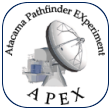Atacama Pathfinder EXperiment APEX OLD pages, check new site Observing time calculator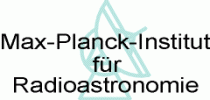APEX TWIKI CONTACT SEARCH HELP NEWS DISCLAIMER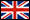# Observing Time Estimate for APEX-2A

On this page we will show how to estimate the observing times with the APEX 345 GHz receiver.

The equivalent system temperature outside the atmosphere is calculated from the expression: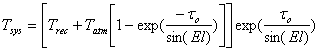(1)

where Trec is the receiver temperature, Tatm is the integrated physical atmospheric temperature (normally about 270 K), τo is the atmospheric zenith opacity and El the elevation of the source. Table 1 lists typical values of the atmospheric zenith opacity at Chajnantor. Eq. (1) provides only a rough estimate (the atmospheric transmission page provides more accurate values), but good enough for most applications. The fact that the telescope also picks up some signal from the ground is not taken into account.

 ν [GHz] 290 345 358 372 τo 0.05 0.09 0.12 0.5

Table 1: Typical zenith opacities at Chajnantor during good conditions (pwv = 0.5 mm)

The rms noise in the spectrum is calculated from: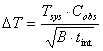(2)

where Cobs is a factor depending on the observing method used, B is the noise bandwidth per channel of the spectrometer and tint the integration time ON source. C is equal to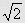for switching and position switching (because noise is added when the OFF spectrum is subtracted from the ON spectrum) and 1 for frequency switching. Solving for tint in Eq. (2), we get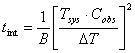(3)

As an example we will now calculate the integration time necessary in order to obtain an r.m.s. noise of 0.1 K with the FFTS bandwidth of 1 GHz at 345 GHz using the various observing methods. The elevation of the source will be assumed to be 60° and the zenith opacity at 345 GHz is about 0.09 (Table 1). The receiver temperature as a function of frequency is shown in Fig. 1 and is about 70 K at 345 GHz. This gives Tsys = 106 K which corresponds to a SSB value of 212 K. For beam and position switching Cobs =and we want to obtain a ΔT of 0.1 K which gives tint = 1.2 min. For beam and position switching this value has to be multiplied by a factor depending on the time spent in the OFF position and an overhead time which includes telescope movements and calibrations. We will assume a value of 3.2 for this factor. The total time for the observation is thus 3.8 min.

The estimated rms noise values should of course be compared with the expected signal intensity. The main beam and moon efficiencies of APEX at 345 GHz are expected to be 0.85 and 1.0, respectively. Thus, a CO(3-2) line with a brightness temperature of 10 K would give an intensity of 10 * 0.85 = 8.5 K, if the source just fills the main beam, or 10 * 0.9=9 K, if the source is extended.